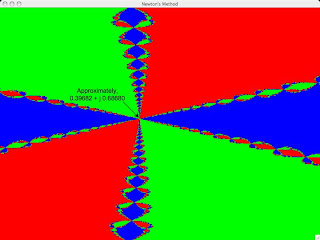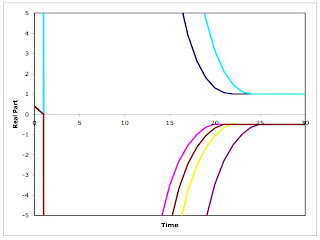## Sunday, September 12, 2010

### Nonergodicity And The Butterfly Effect

1.0 Introduction
Cosma Shalizi says, "It is not true that ergodicity is incompatible with sensitive dependence on initial conditions." This poses some questions for me: Can I give an example of a non-ergodic process that also exhibits sensitive sensitive dependence on initial conditions? Can I give an example of an ergodic process that exhibits sensitive dependence on initail conditions?

This post answers the former question. I consider Newton's method for finding roots of unity in the complex plane. The latter question is probably more important for Cosma's assertion. For now, I cite the Lorenz equations as an example of an ergodic process with the desired sensitive dependence.

Cosma's assertion, "It is not true that non-stationarity is a sufficient condition for non-ergodicity," directly contradicts Paul Davidson. I do not address that contradiction here.

2.0 Newton's Method
Newton's method is an algorithm for finding the zeros of a function. In this post, I illustrate the method with the function:
F(z) = z3 - 1,
where z is a complex number. A complex number can be written as a two-element vector:
z = [x, y]T = x + jy
where j is the square root of negative one. (I've been hanging around electrical engineers.) Likewise, one can consider the function F as a vector of two elements:
F(z) = [f1(z), f2(z)]T
The first component maps the real and imaginary parts of the argument to the real part of the function value:
f1(z) = x3 - 3xy2 - 1
The second component maps to the imaginary component of the function value:
f2(z) = y (3x2 - y2)

Newton's method is for numerically finding a solution to the following equation:
F(z) = 0
In my case, one is searching for the cube roots of unity. The method is an iterative method. An initial guess is refined until successive guesses are close enough together that one is willing to accept that the method has converged. A guess is refined by taking a linear approximation to the function at the guess. That guess is refined by solving for the zero of that linear approximation. The zero is the next iteration.

The derivative of a function, when evaluated at the current iterate, provides the linear approximation. The Jacobian is the two-dimensional equivalent of the derivative. The Jacobian, J, is a matrix with the following elements:
Ji, 1([x, y]T) = dfi([x, y]T)/dx, i = 1, 2.
Ji, 2([x, y]T) = dfi([x, y]T)/dy, i = 1, 2.

Newton's method is specified by the following iterative equation:
zn + 1 = zn - J-1(zn) * F(zn)

3.0 Numeric Explorations
Figure 1 shows a coloring of the plane based on the application of Newton's method. Each point in the plane can be selected as an initial point. The method is applied, and the point is colored according to which of the three cube roots of unity to which the method converges. Figure 2 shows an enlargement of the region around the indicated point in the northeast of Figure 1. Notice the fractal nature of the regions of convergence.Figure 1: Fractal Basins of Attraction for Newton's MethodFigure 2: An Enlargement of These Fractal Basins

To exhibit sensitive conditions on initial conditions, I wanted to find nearby points whose trajectory diverges under this dynamical process. Table 1 lists six points selected from Figure 2. They fall into three groups, depending on which root they converge to. I claim that one can find at least three distinct points such that each pair is as close as one wants that each converge to a seperate root.
 Initial Guess Limit Point 0.3899 + j 0.6871 1 0.3938 + j 0.6780 (-1/2) - j (31/2)/2 0.3986 + j 0.6811 (-1/2) + j (31/2)/2 0.4010 + j 0.6868 1 0.3980 + j 0.6908 (-1/2) - j (31/2)/2 0.3943 + j0.6949 (-1/2) + j (31/2)/2
Figure 3 and 4 display the trajectories of the six points selected for Table 1. Apparently the function is very shallow in this region. I had not realized before these explorations that these sorts of trajectories go so far from the origin before returning to converge to a root on the unit circle.Figure 3: Real Part of Some Time Series From Newton's MethodFigure 4: Imaginary Part of Some Time Series From Newton's Method

4.0 Conclusions
Cosma provides this definition, among others, of an ergodic process:
"A ... process is ergodic when ... (almost) all trajectories generated by an ergodic process belong to a single invariant set, and they all wander from every part of that set to every other part..."
This definition is appropriate for both deterministic and stochastic processes.

The three roots of unity constitute the non-wandering (invariant) set for the dynamical system created by the above application of Newton's method. A trajectory that has converged to one of the roots does not wander to any other root. So the process is non-ergodic. Yet which root a process converges to is crucially dependent on the initial conditions. A small variation in the initial conditions leads to a long-term divergence in trajectories. This is especially evident because of the fractal structure of the basins of attraction of the three roots.

I think of the above as close to recreational mathematics. I have not tied the above example into any economics model. Common neoclassical models, such as the Arrow-Debreu model of general equilibrium, fail to tie dynamics down. I find it difficult to see how one who has absorbed this fact and understands the mathematics of dynamical systems can find credible much orthodox teaching in economics.

Cosma Shalizi said...

And, indeed, talking about fixed points without worrying about dynamics is one of the reasons I find much of orthodox economics quite incredible.

BruceMcF said...

Further, in the actual problem domain, the set of possible trajectories evolves in historical time, and that evolution is dependent in part on the trajectory.

We know from empirical observation that economic development in the real world contradicts the definition of an ergodic process ... working out precisely how little of what we know about the economy can be retained and still reject the definition is of intellectual interest, but its more in the nature of a mathematical question than a scientific one.Anonymous said...

You claim dynamics are searching through nonequilibrium price-quantity pairs for equilibrium. Nobody uses it this way -- dynamics are part of the equilibrium. Since you can't prove that supply and demand aren't always equal, your emperor has no clothes.